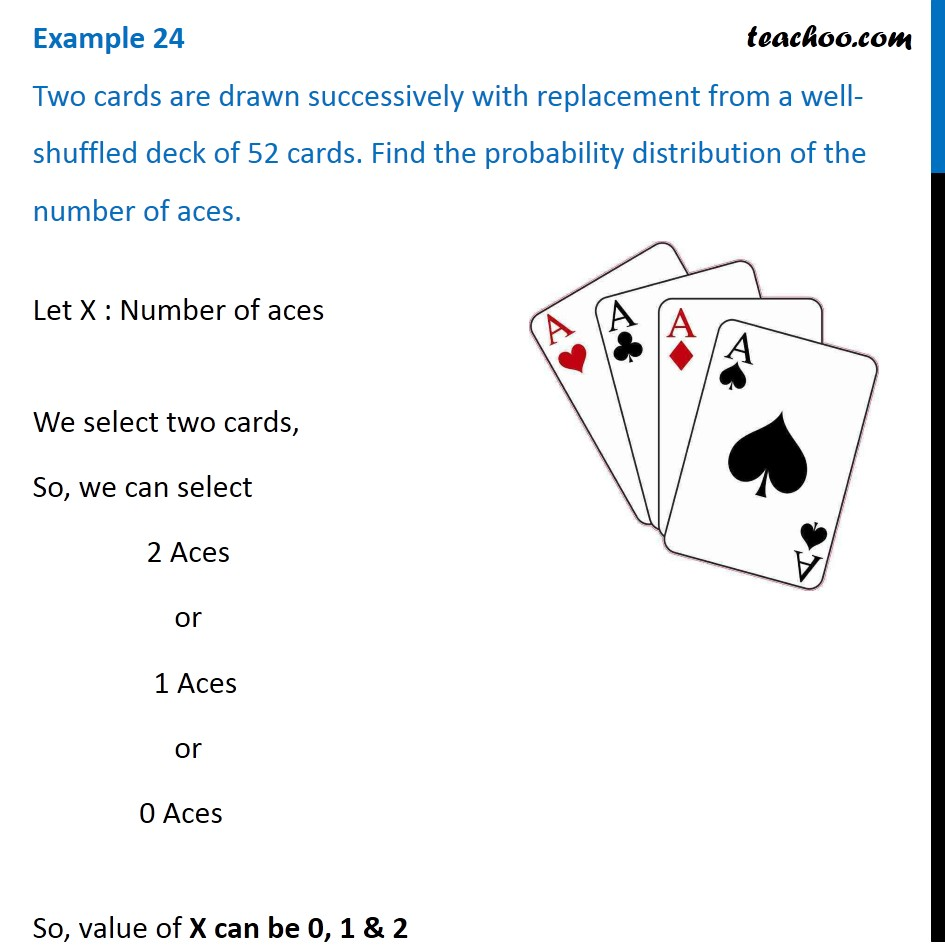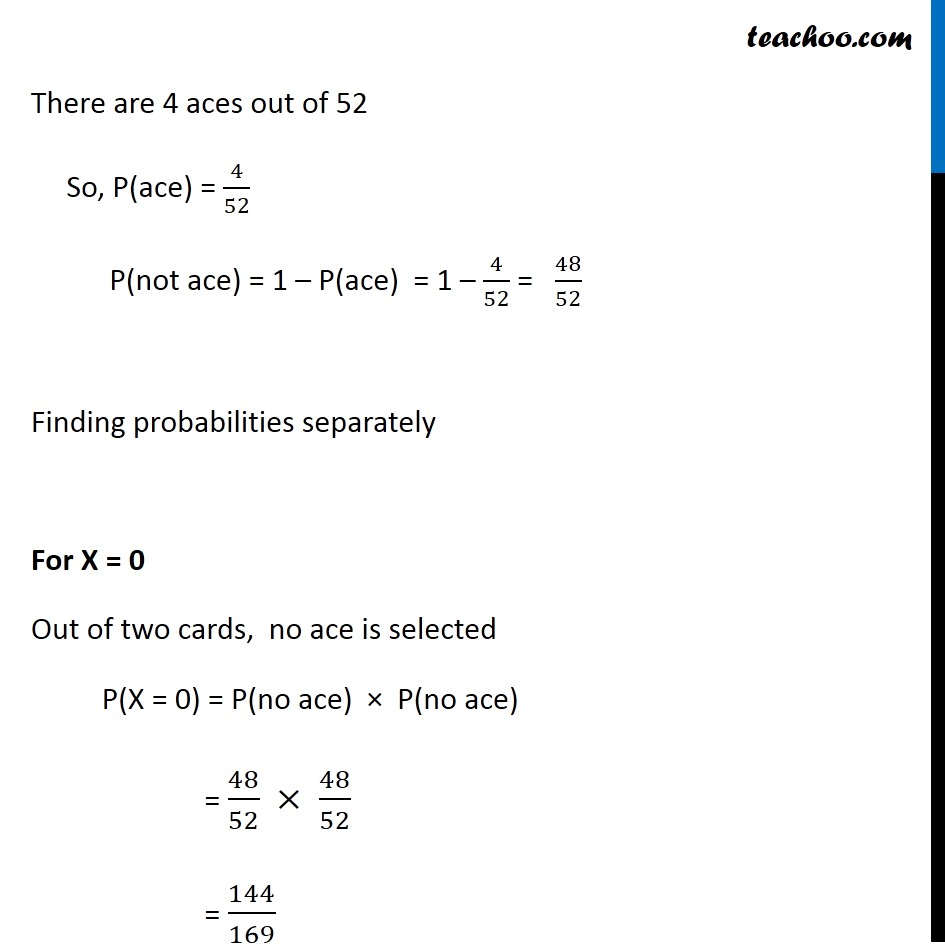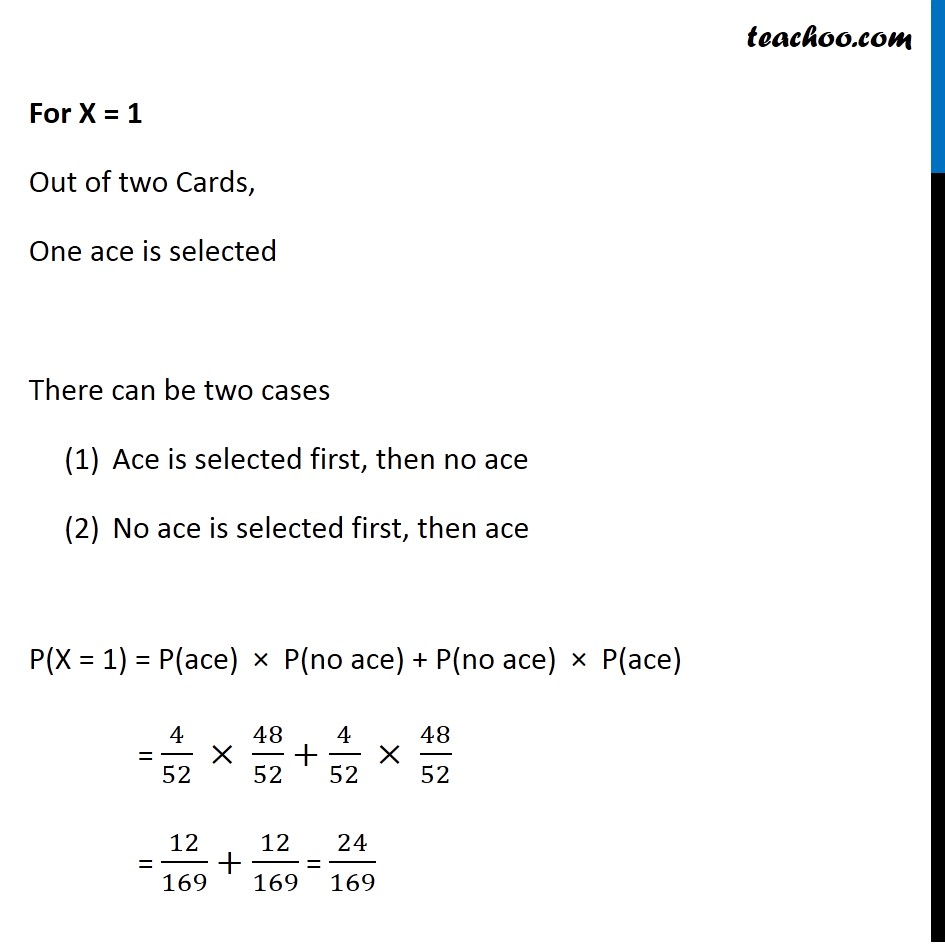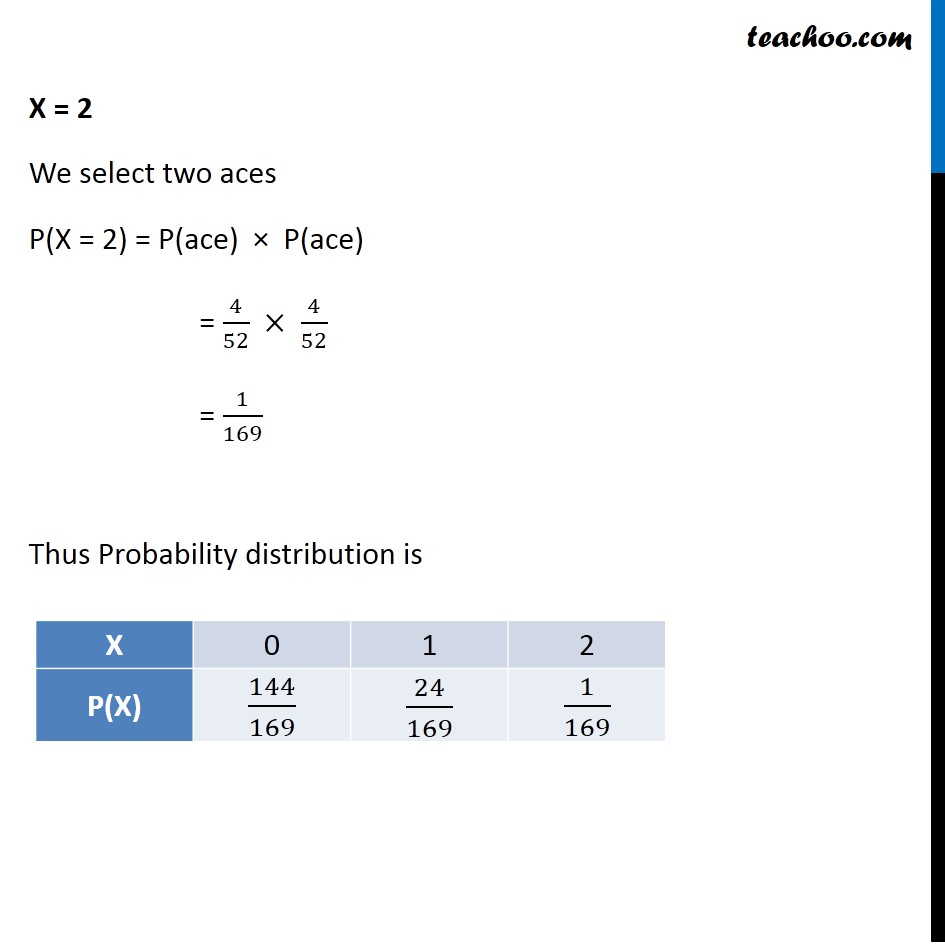Probability distribution

Chapter 13 Class 12 Probability (Term 2)
Concept wise### Transcript

Example 24 Two cards are drawn successively with replacement from a well-shuffled deck of 52 cards. Find the probability distribution of the number of aces. Let X : Number of aces We select two cards, So, we can select 2 Aces or 1 Aces or 0 Aces So, value of X can be 0, 1 & 2 There are 4 aces out of 52 So, P(ace) = 4/52 P(not ace) = 1 – P(ace) = 1 – 4/52 = 48/52 Finding probabilities separately For X = 0 Out of two cards, no ace is selected P(X = 0) = P(no ace) × P(no ace) = 48/52 × 48/52 = 144/169 There are 4 aces out of 52 So, P(ace) = 4/52 P(not ace) = 1 – P(ace) = 1 – 4/52 = 48/52 Finding probabilities separately For X = 0 Out of two cards, no ace is selected P(X = 0) = P(no ace) × P(no ace) = 48/52 × 48/52 = 144/169 For X = 1 Out of two Cards, One ace is selected There can be two cases Ace is selected first, then no ace No ace is selected first, then ace P(X = 1) = P(ace) × P(no ace) + P(no ace) × P(ace) = 4/52 × 48/52+4/52 × 48/52 = 12/169+12/169 = 24/169 X = 2 We select two aces P(X = 2) = P(ace) × P(ace) = 4/52 × 4/52 = 1/169 Thus Probability distribution is